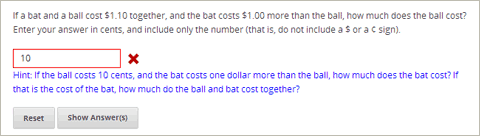# 7.30. Problem with Adaptive Hint¶

A problem with an adaptive hint evaluates a student’s response, then gives the student feedback or a hint based on that response so that the student is more likely to answer correctly on the next attempt. These problems can be text input problems or multiple choice problems.## 7.30.1. Create a Problem with an Adaptive Hint¶

To create the above problem:

1. In the unit where you want to create the problem, click Problem under Add New Component, and then click the Advanced tab.
2. Click Problem with Adaptive Hint.
3. In the component that appears, click Edit.
4. In the component editor, replace the example code with the code below.
5. Click Save.
<problem>
<text>
<script type="text/python" system_path="python_lib">
def test_str(expect, ans):
print expect, ans
ans = ans.strip("'")
ans = ans.strip('"')
return expect == ans.lower()

def hint_fn(answer_ids, student_answers, new_cmap, old_cmap):
aid = answer_ids
ans = str(student_answers[aid]).lower()
print 'hint_fn called, ans=', ans
hint = ''
if '10' in ans:
hint = 'If the ball costs 10 cents, and the bat costs one dollar more than the ball,
how much does the bat cost? If that is the cost of the bat, how much do the ball and
bat cost together?'
elif '.05' in ans:
hint = 'Make sure to enter the number of cents as a whole number.'

if hint:
hint = "&lt;font color='blue'&gt;Hint: {0}&lt;/font&gt;".format(hint)
new_cmap.set_hint_and_mode(aid,hint,'always')
</script>
<p>If a bat and a ball cost $1.10 together, and the bat costs$1.00 more than the
ball, how much does the ball cost? Enter your answer in cents, and include only
the number (that is, do not include a \$ or a ¢ sign).</p>
<p>
<customresponse cfn="test_str" expect="5">
<textline correct_answer="5" label="How much does the ball cost?"/>
<hintgroup hintfn="hint_fn"/>
</customresponse>
</p>
</text>
</problem>


## 7.30.2. Problem with Adaptive Hint XML¶

### 7.30.2.1. Template¶

<problem>
<text>
<script type="text/python" system_path="python_lib">
def test_str(expect, ans):
print expect, ans
ans = ans.strip("'")
ans = ans.strip('"')
return expect == ans.lower()

def hint_fn(answer_ids, student_answers, new_cmap, old_cmap):
aid = answer_ids
ans = str(student_answers[aid]).lower()
print 'hint_fn called, ans=', ans
hint = ''
if 'incorrect answer 1' in ans:
hint = 'hint for incorrect answer 1'
elif 'incorrect answer 2' in ans:
hint = 'hint for incorrect answer 2'

if hint:
hint = "&lt;font color='blue'&gt;Hint: {0}&lt;/font&gt;".format(hint)
new_cmap.set_hint_and_mode(aid,hint,'always')
</script>
<p>TEXT OF PROBLEM</p>
<p>
<customresponse cfn="test_str" expect="ANSWER">
<textline correct_answer="answer" label="LABEL TEXT"/>
<hintgroup hintfn="hint_fn"/>
</customresponse>
</p>
</text>
</problem>


Note

If the hints that you supply include characters, the letters must be lowercase.

### 7.30.2.2. Tags¶

• <text>: Surrounds the script and text in the problem.
• <customresponse>: Indicates that this problem has a custom response.
• <textline>: Creates a response field in the LMS where the student enters a response.
• <hintgroup>: Specifies that the problem contains at least one hint.

Tag: <customresponse>

Attributes

(none)

Children

• <textline>
• <hintgroup>

Tag: <textline>

Attributes

Attribute Description
label (required) Contains the text of the problem.
size (optional) Specifies the size, in characters, of the response field in the LMS.
hidden (optional) If set to “true”, students cannot see the response field.
correct_answer (optional) The answer to the problem. To supply a correct_answer value that includes letters, all letters must be lowercase. (Students’ responses to the problem are not case sensitive. They can contain both uppercase and lowercase letters.)

Children

(none)

Tag: <hintgroup>

Attributes

Attribute Description
hintfn Must be set to hint_fn (that is, the tag must appear as <hintgroup hintfn="hint_fn"/>).